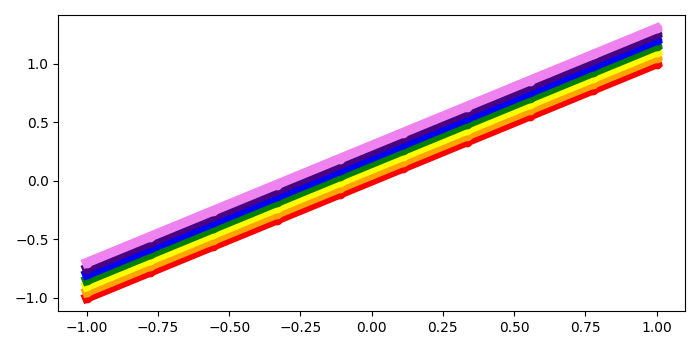# How to plot a multi-colored line, like a rainbow using Matplotlib?

To plot multi-colored lines, like a rainbow, we can create a list of seven rainbow colors (VIBGYOR).

## Steps

• Create x for data points using numpy.

• Create a list of colors (rainbow VIBGYOR).

• Iterate in the range of colors list length.

• Plot lines with and y(x+i/20) using plot() method, with marker=o, linewidth=7 and colors[i] where i is the index.

• To display the figure, use show() method.

## Example

import numpy as np
from matplotlib import pyplot as plt
plt.rcParams["figure.figsize"] = [7.00, 3.50]
plt.rcParams["figure.autolayout"] = True
x = np.linspace(-1, 1, 10)
colors = ["red", "orange", "yellow", "green", "blue", "indigo", "violet"]
for i in range(len(colors)):
plt.plot(x, x+i/20, c=colors[i], lw=7, marker='o')
plt.show()

## Output